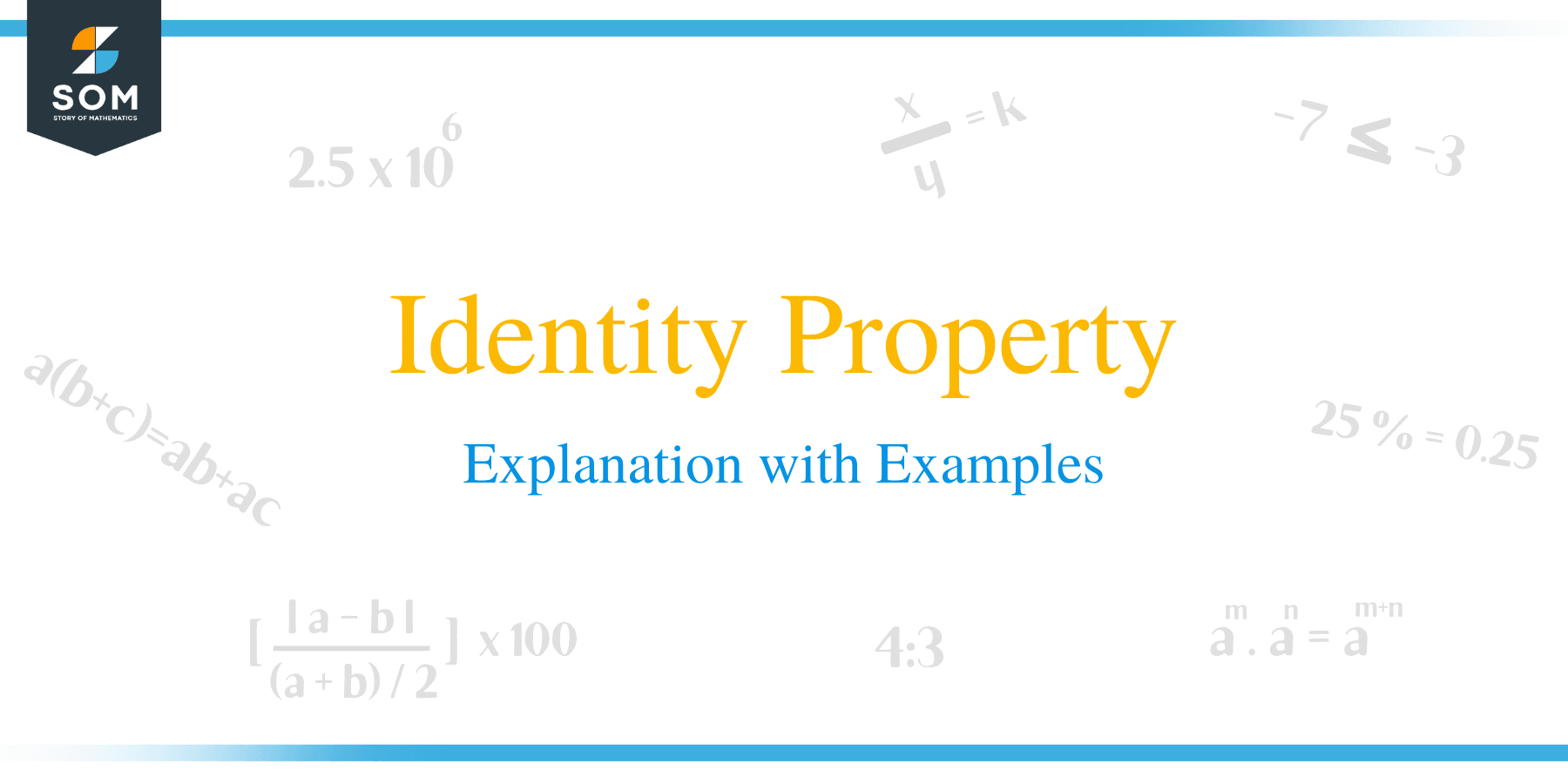# Identity Property – Explanation with Examples

##What is Identity Property?

Real numbers are an ordered set of numbers that possess unique properties. The basic properties are commutative, associative, distributive, and identity. An identity property is a property that applies to a group of numbers in the form of a set. It cannot be applied to any individual number only.

It is named identity property because when applied to a number, the number keeps its ‘identity.’ The identity property is true for all arithmetic operations.The identity property of addition is that when a number n is added to zero, the result is the number itself i.e.

n + 0 = n

Zero is called an additive identity and it can be added to any real number without changing its value. Here are the few examples of identity property of addition,

3 + 0 = 3          (Positive Integers)

-3 + 0 = -3       (Negative Integers)

4/5 + 0 = 4/5    (Fractions)

0.5 + 0 = 0.5    (Decimals)

x + 0 = x          (Algebraic notation)

This property holds true for subtraction as well because subtracting 0 from any number equals the number itself. Therefore, 0 is also called a subtractive identity.

## Identity Property of MultiplicationThe identity property of multiplication is that when a number n is multiplied by one, the result is the number itself i.e.

n × 1 = n

One is called the multiplicative identity, and it can be multiplied with any real number without changing its value. Here are a few examples of identity property of multiplication,

3 × 1 = 3          (Positive Integers)

-3 × 1 = -3       (Negative Integers)

4/5 × 1 = 4/5    (Fractions)

0.5 × 1 = 0.5    (Decimals)

x × 1 = x          (Algebraic notation)

This property holds true for division as well because dividing any number by 1 equals the number itself. Therefore, 1 is also called divisive identity.

### Practice Questions

1. Which of the following numbers will make the equation, $\_\_\_ \times -9 = -9$, true.

2. Which of the following numbers will make the equation, $-2 \times \_\_\_ = -2$, true.

3. Which of the following numbers will make the equation, $\_\_\_ \times 1= 0.981$, true.

4. Which of the following numbers will make the equation, $\left(\dfrac{7}{3} + \dfrac{2}{3}\right) \times 1= \_\_\_$, true.

5. Which of the following numbers will make the equation, $\_\_\_ \times 1= \dfrac{99}{100}$, true.

6. Which of the following numbers will make the equation, $(85 – 23 + 32) \times 1=\_\_\_$, true.

7. Jane can place $12$ eggs per crate. How many eggs must be there in $1$ crate?

8. There are $8$ rooms in a house. Each room has $1$ bed. How many beds are there in total?

9. In a country, each State has one minister. How many ministers are there, if there are $20$ States in that country?

10. Sam works in a coffee shop and earns $\$20$per hour. How much does he earn on a particular day when he works for$1\$ hour only?